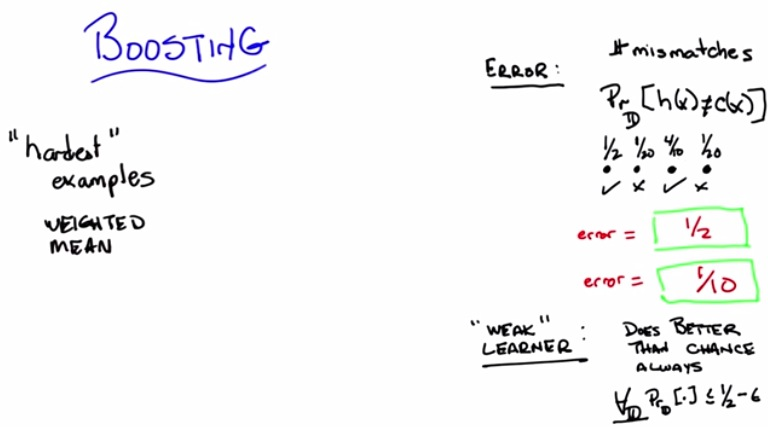• So instead of taking uniformly randomly subsets of data, take "hardest examples" from it
• And, instead of combine just the mean, combine the "weighted mean"
• So what's "Hardest examples"? what's "weighted mean"?
• Earlier, the error, for classifier, is just taking the overall number of mistmatches of the label
• Now the error is as formula on the top right corner.
• Suppose we are giving 4 data points, 2 of them mispredicted. What are the learner probabilty? 1/2
• Suppose there's 1/2 chance of seeing points no. 1, and so forth as listed.
• Then we just taking the  sum of the error, over all the sum of probability. 2 error = 1/20 + 1/20 = 1/10
• So instead of treat all of them normally, treat them based on the higher probability(hardest example).
• that might be the case for weighted mean.
• It's preferable to treat the hardest example more than easiest example.
• Weak learner, is, ignore all the distribution, the prediction is always less half than some of the threshold(epsilon) value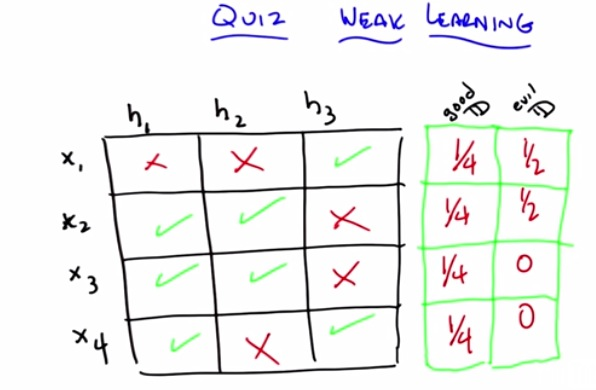• So what's the distribution of all x values, in such a way, that h1,h2.h3 satisfy the condition? green correctly predict the value, red mistmatche the value.
• good D, have to sum up to one, as there's only 4 points.
• now if it's true for normal distribution, than the weight must be average.
• If it's normal distribution (0.5,0.5,0,0) than h1 is better than chance(predict 0.75 > 0.5 chance). h1 is better one eventhough 2 other is just 0.5
• As long there's one hypothesis better, than we can safely assume this is a good distribution.
• Now for evil distribution (0.5,0.5,0,0) this makes h1,h2,h3 just 0.5 chance to predict (in predicting x1 and x2). And this makes it such evil distribution that all the hypothesis couldn't do better than chance.
• Not that we also don't have  a weak learner in this case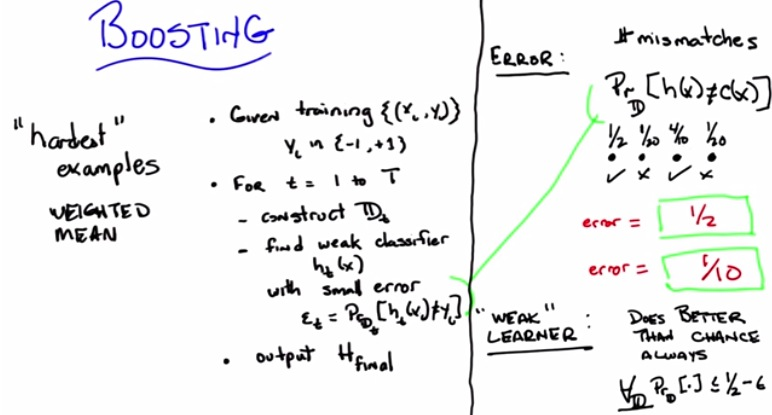• So this is overall the boosting algorithm
• We have training set, given x, and y is the binary classifier output, - not in class, + in class
• Iterate the step to T with t as iterator.
• Construct some distribution (taught later)
• Find weak classfier with low error.
• Combine all hypothesis of t steps into one final (taught later)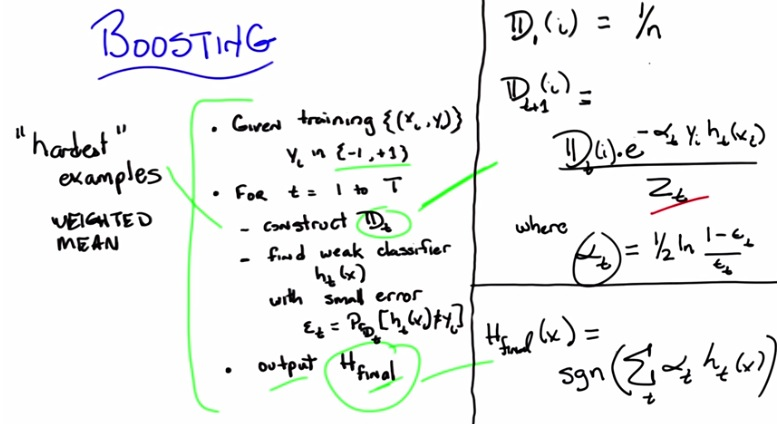• So as usual as we don't know what's distribution, we put init normal distribution (uniform distribution)
• Then, we create next distribution. based on the equation above.
• alpha acts like some kind of learning rate. positive number in range 0 to 1
• y.h produce positive if agree one another (-1x-1) or (1x1) otherwise negative
• Now what will happen if both H and y are agree? Depends on the alpha sub t
• Alpha sub t is half of natrual log, times how well we are doing (1-eps) over the error(eps). This is the error threshold that we've been talking
• e produce negative/positive range from 0 to 1
• The whole result is distribution will put more weight to the one that mismatched, and less weight to the one that matched.
• Combine with all the distribution, all alpha, and all hypothesis create one final hypothesis
• This is the overall boosting function.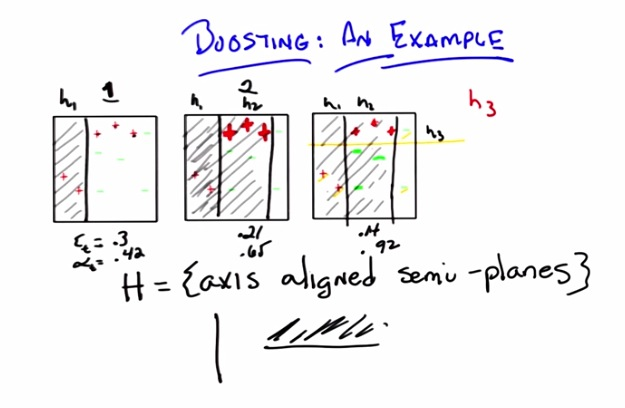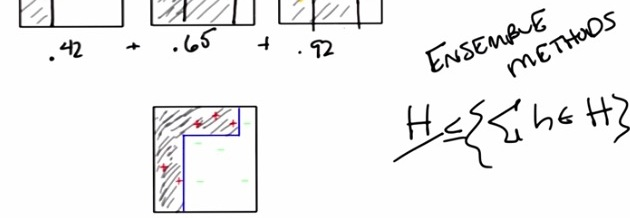• So these are the step examples of boosting algorithm
• there's three litlle boxing showing each steps of boosting algorithm
• First it will perform some axis separated lines (vertical/horizontal) to split the area
• First paragraph have error 0.3, alpha 0.42. We see that every steps, error decreases, and alpha increases(put more and more weight)
• In first step, over 10 data points, the learner missclassify 3, so the error is 3/10 = 0.3. Feel free to calculate the alpha based on formula.
• In second step, 3 plus that get missclassfied has put more weight(drawn by more border).That way the learner gets to know not to missclasify those 3 (prioritize).Then it split the vertical axis more to the right.That split in the middle. and we can see that it missclasify 3 of the data points. The error should not be 0.3, since we have changing the weighted distribution. and get 0.21
• The third step, 3 green missclasify get more weight, even more than 3 previous red. with all remaining points (circled by yellow) even smaller and smaller(because it doesn't get any weight).It split with any missclasfied, then it stop.
• The result will be combining all the axis separator, and can missclasfy correctly.
• This ensemble method will be at least or less complicated than sometimes other classfier.
• This is same as NN, where we take each step, local weighted classifier.

• So this is the whole point of all boosting algorithm
• What are the main advantage over the others?
• Because it's main concern is to be better and better on all the previous wrong missclasfiy.
• Suppose we know nothing of the distribution (normal distribution). What it does is, perform distribution everytime, so all the wrong examples becomes more matters, until we have finished, doesn't have all the wrong example.
• What if we cycle back and forth? over the three, first predict first two right, then predict last two right?
• Boosting force the learner to be better and better, not just take all examples as normal distribution, but more and more weight to the one that have been wrong.Over all the three examples and two step process, the middle always goes right. So the weight of middle becomes smaller and practically don't get attention. The boosting focus on the first and the last. and everytime it does the step. The weight becomes more and more weight to the wrong one, until one of the two have becomes much more weight than the other, and therefore classify correclty.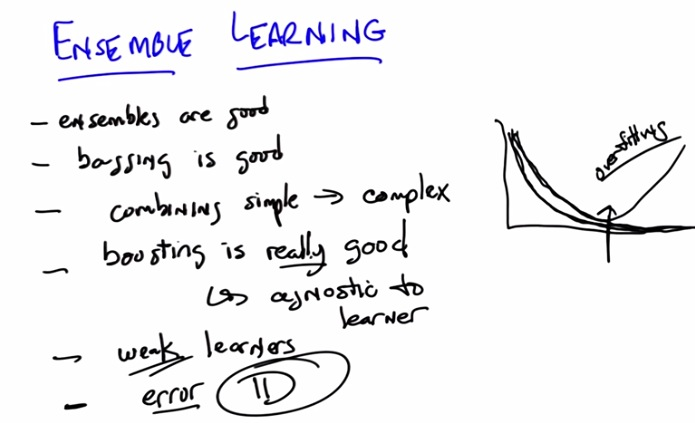• This is the summary, and what do we know? turns out boosting have beat the danger of overfitting, the danger that most machine learning algorithm have